# 如何实现物体透视效果 – 正交和透视投影矩阵

## theme: qklhk-chocolate highlight: an-old-hope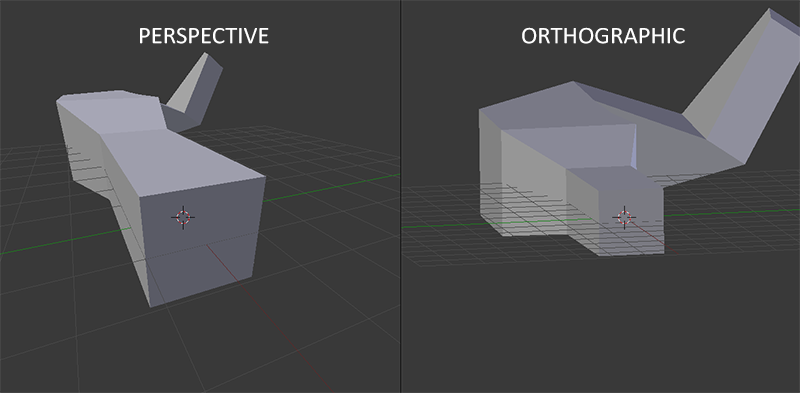## 正交投影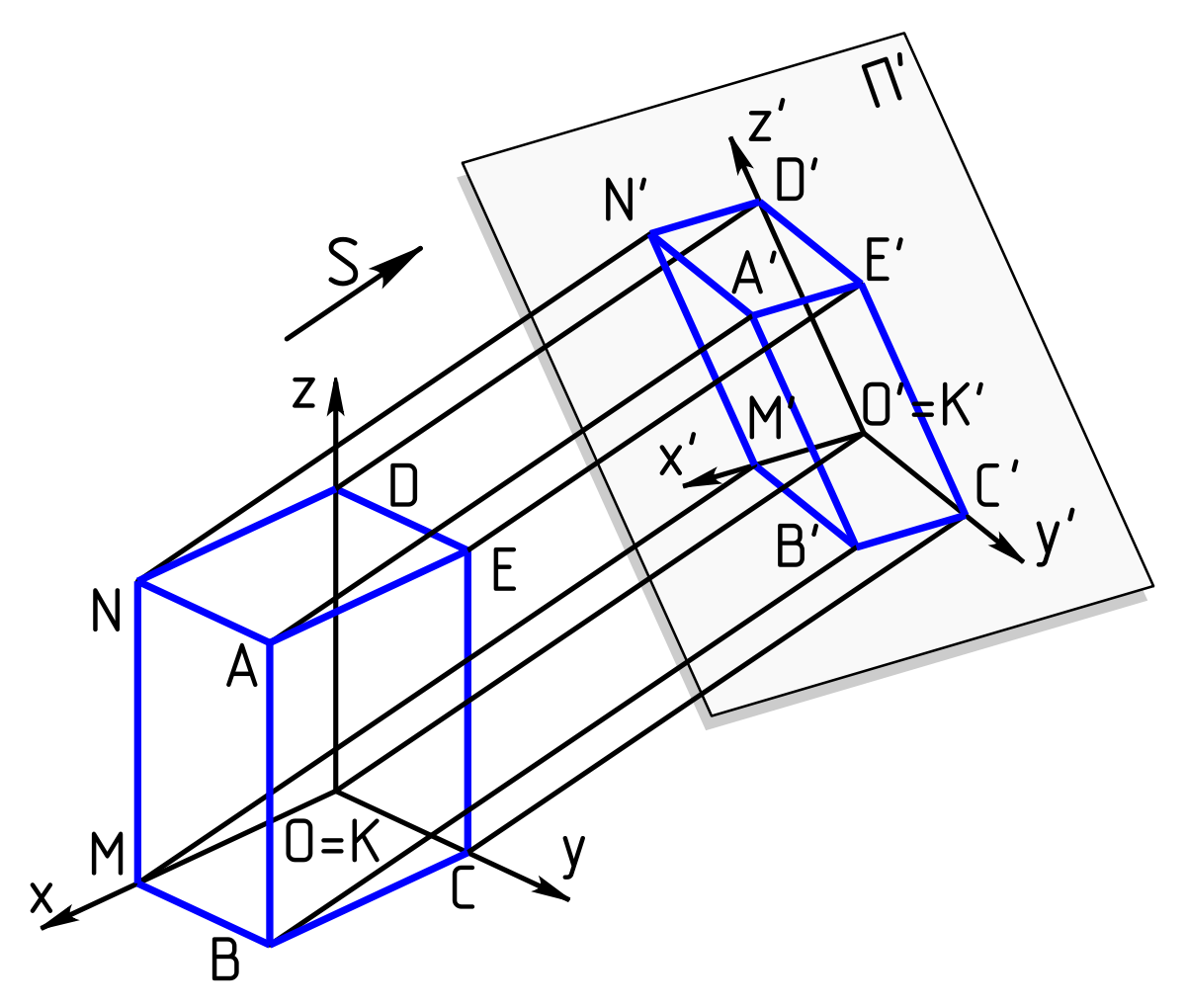$$\begin{bmatrix} 1 & 0 & 0 & 0 \ 0 & 1 & 0 & 0 \ 0 & 0 & 0 & 0 \ 0 & 0 & 0 & 1 \end{bmatrix}$$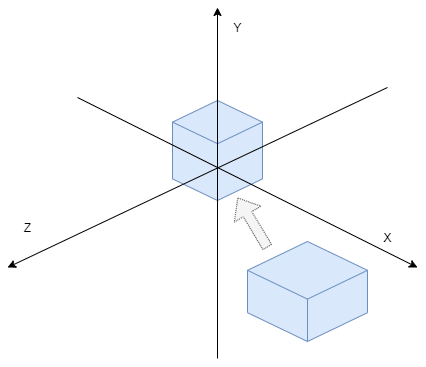$$X_{ndc}=S*X_{eye}+D$$

$$X_{ndc}=\frac{2}{right-left}*X_{eye}+D$$

$$1=\frac{2}{right-left}*right+D$$

$$D=-\frac{right+left}{right-left}$$

$$Y_{ndc}=\frac{2}{top-bottom}*Y_{eye}-\frac{top+bottom}{top-bottom}$$

$$Z_{ndc}=-\frac{2}{far-near}*Z_{eye}-\frac{far+near}{far-near}$$

$$\begin{bmatrix} \frac{2}{r-l} & 0 & 0 & -\frac{r+l}{r-l} \ 0 & \frac{2}{t-b} & 0 & -\frac{t+b}{t-b} \ 0 & 0 & -\frac{2}{f-n} & -\frac{f+n}{f-n} \ 0 & 0 & 0 & 1 \end{bmatrix}$$

class Mat4 {
static ortho(left, right, bottom, top, near, far, out = []) {
const lr = 1 / (left - right);
const bt = 1 / (bottom - top);
const nf = 1 / (near - far);
out = -2 * lr;
out = 0;
out = 0;
out = 0;
out = 0;
out = -2 * bt;
out = 0;
out = 0;
out = 0;
out = 0;
out = 2 * nf;
out = 0;
out = (left + right) * lr;
out = (top + bottom) * bt;
out = (far + near) * nf;
out = 1;
return out;
}
}


const gl = createGl()

const program = createProgramFromSource(gl, 
attribute vec4 aPos;
uniform mat4 uMat;

void main() {
gl_Position = uMat * aPos;
}
, 
precision highp float;

void main() {
gl_FragColor = vec4(gl_FragCoord.zzz, 1);
}
)

const box = createBox()
// { index: { value: [], size: 1 }, position: { value: [], size: 3 } }

const indexBuffer = gl.createBuffer()
gl.bindBuffer(gl.ELEMENT_ARRAY_BUFFER, indexBuffer)
gl.bufferData(gl.ELEMENT_ARRAY_BUFFER, box.index.value, gl.STATIC_DRAW)

const [posLoc] = createAttrBuffer(gl, program, 'aPos', box.position.value)
gl.vertexAttribPointer(posLoc, 3, gl.FLOAT, false, 0, 0)
gl.enableVertexAttribArray(posLoc)

const camera = new Camera()
camera.position.x = camera.position.y = camera.position.z = 0.5
camera.lookAt([0, 0, 0])
const matLoc = gl.getUniformLocation(program, 'uMat')
gl.uniformMatrix4fv(matLoc, false, Mat4.multiply(
Mat4.ortho(-2, 2, -2, 2, -2, 2),
camera.viewMatrix
))

gl.enable(gl.DEPTH_TEST)
gl.enable(gl.CULL_FACE)
gl.clearColor(0, 0, 0, 0)
gl.clear(gl.COLOR_BUFFER_BIT | gl.DEPTH_BUFFER_BIT)
gl.drawElements(gl.TRIANGLES, box.index.value.length, gl.UNSIGNED_SHORT, 0)

}

function createProgramFromSource(gl, vertex, fragment) {
const program = gl.createProgram()
gl.useProgram(program)
return program
}

function createAttrBuffer(gl, program, attr, data) {
const location = gl.getAttribLocation(program, attr)
const buffer = gl.createBuffer();
gl.bindBuffer(gl.ARRAY_BUFFER, buffer)
gl.bufferData(gl.ARRAY_BUFFER, data, gl.STATIC_DRAW)
return [location, buffer]
}


https://code.juejin.cn/pen/7168488413334044685

## 透视投影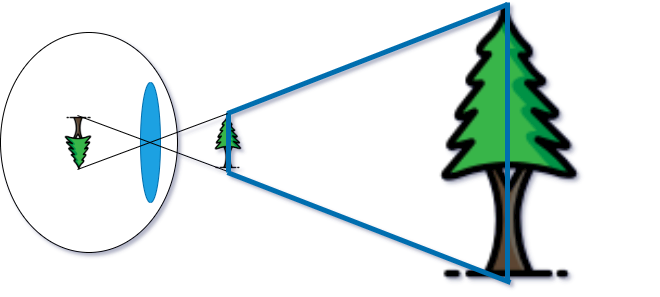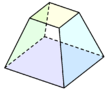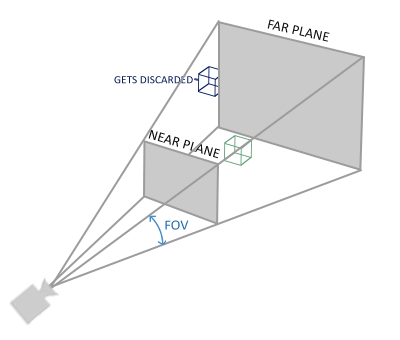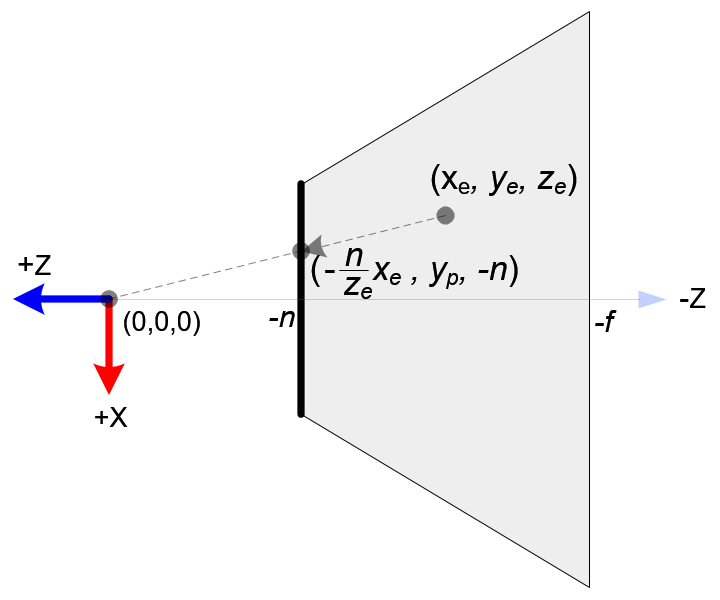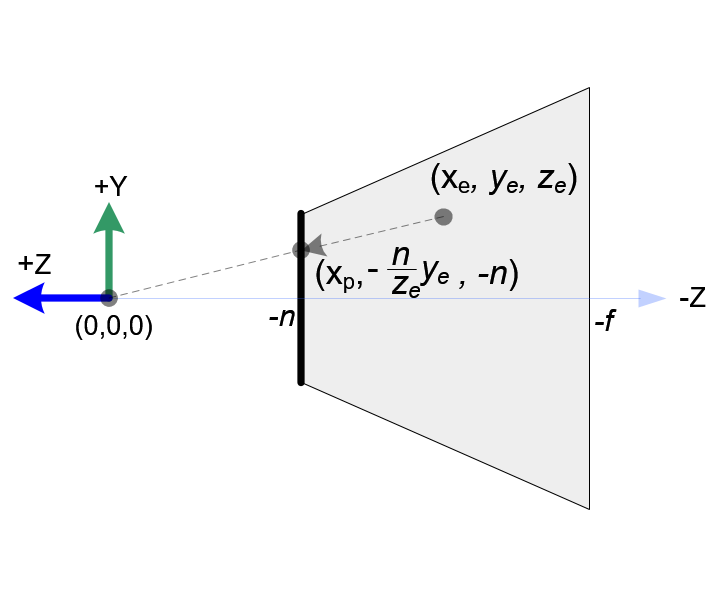$$\begin{bmatrix} n & 0 & 0 & 0 \ 0 & n & 0 & 0 \ ? & ? & ? & ? \ 0 & 0 & -1 & 0 \end{bmatrix}$$

$$\begin{bmatrix} n & 0 & 0 & 0 \ 0 & n & 0 & 0 \ 0 & 0 & A & B \ 0 & 0 & -1 & 0 \end{bmatrix}$$

\begin{aligned} [0, 0, A, B] \cdot [x,y,-n,1] &= -n^2 \ A*-n+B&=-n^2 \end{aligned}

\begin{aligned} [0,0,A,B] \cdot [0,0,-f,1] &= -f^2 \ A*-f+B=-f^2 \end{aligned}

\begin{aligned} A&=n+f\ B&=nf \end{aligned}

$$\begin{bmatrix} n & 0 & 0 & 0 \ 0 & n & 0 & 0 \ 0 & 0 & n+f & nf \ 0 & 0 & -1 & 0 \end{bmatrix}$$

\begin{aligned} M_p&=M_o * M_{p \to o} \ &=\begin{bmatrix} \frac{2}{r-l} & 0 & 0 & -\frac{r+l}{r-l} \ 0 & \frac{2}{t-b} & 0 & -\frac{t+b}{t-b} \ 0 & 0 & -\frac{2}{f-n} & -\frac{f+n}{f-n} \ 0 & 0 & 0 & 1 \end{bmatrix} \begin{bmatrix} n & 0 & 0 & 0 \ 0 & n & 0 & 0 \ 0 & 0 & n+f & nf \ 0 & 0 & -1 & 0 \end{bmatrix} \ &=\begin{bmatrix} \frac{2n}{r-l} & 0 & \frac{r+l}{r-l} & 0 \ 0 & \frac{2n}{t-b} & \frac{t+b}{t-b} & 0 \ 0 & 0 & -\frac{f+n}{f-n} & -\frac{2nf}{f-n} \ 0 & 0 & -1 & 0 \end{bmatrix} \end{aligned}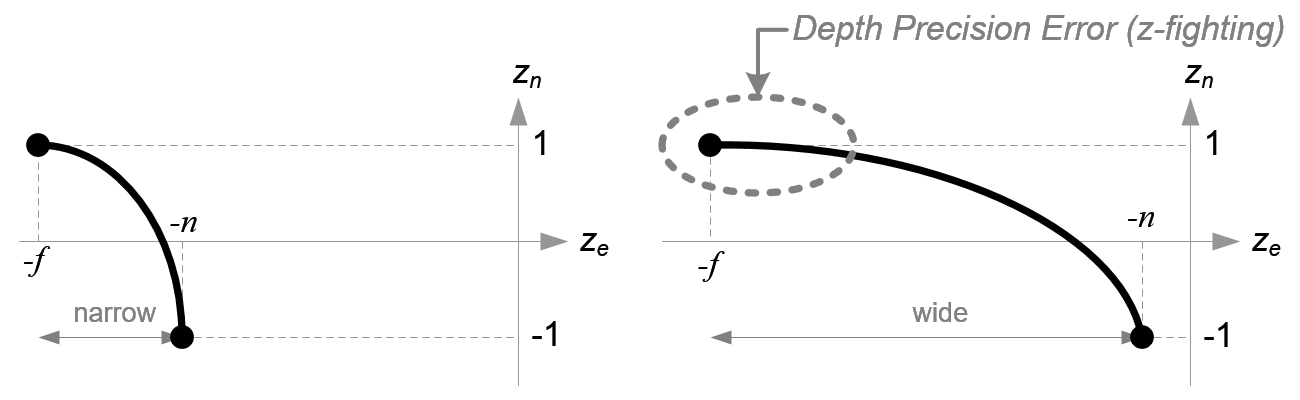### 视野和宽高比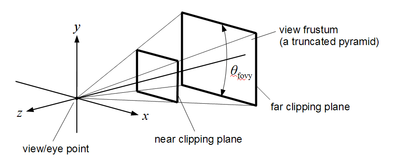• fovy 是 Y 轴的 field of view 表示平截头体顶部和底部的角度，这个角度越大看到的范围越大，物体也会越小。
• aspectaspect ratio 表示视口的宽高比，也就是上方近裁切面的宽高比。
• near 是近平面
• far 是远平面

$$r+l=0 \ r-l=2r \ t+b=0 \ t-b=2t$$

$$\begin{bmatrix} \frac{n}{f} & 0 & 0 & 0 \ 0 & \frac{n}{t} & 0 & 0 \ 0 & 0 & -\frac{f+n}{f-n} & -\frac{2nf}{f-n} \ 0 & 0 & -1 & 0 \end{bmatrix}$$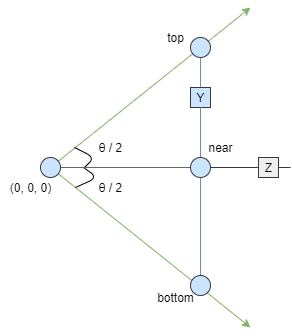$$tab(\frac{\theta}{2}) = \frac{t}{n}$$
$$aspect = \frac{2r}{2t} = \frac{r}{t}$$
$$\frac{n}{t}=\frac{1}{tan(\frac{\theta}{2})}$$
$$\frac{n}{r}=\frac{1}{\frac{r}{t}*\frac{t}{n}} = \frac{1}{aspect * tan(\frac{\theta}{2})}$$

$$\begin{bmatrix} \frac{1}{aspect * tan(fovy / 2)} & 0 & 0 & 0 \ 0 & \frac{1}{tan(fovy / 2)} & 0 & 0 \ 0 & 0 & -\frac{f+n}{f-n} & -\frac{2nf}{f-n} \ 0 & 0 & -1 & 0 \end{bmatrix}$$

class Mat4 {
static perspective(fovy, aspect, near, far, out = []) {
const f = 1 / Math.tan(fovy / 2);
out = f / aspect;
out = 0;
out = 0;
out = 0;
out = 0;
out = f;
out = 0;
out = 0;
out = 0;
out = 0;
out = -1;
out = 0;
out = 0;
out = 0;
if (far != null && far !== Infinity) {
const nf = 1 / (near - far);
out = (far + near) * nf;
out = 2 * far * near * nf;
} else {
out = -1;
out = -2 * near;
}
return out;
}
}


### 渲染立方体

const gl = createGl()

const program = createProgramFromSource(gl, 
attribute vec4 aPos;
uniform mat4 uMat;

void main() {
gl_Position = uMat * aPos;
}
, 
precision highp float;

void main() {
gl_FragColor = vec4(gl_FragCoord.zzz, 1);
}
)

const box = createBox()
// { index: { value: [], size: 1 }, position: { value: [], size: 3 } }

const indexBuffer = gl.createBuffer()
gl.bindBuffer(gl.ELEMENT_ARRAY_BUFFER, indexBuffer)
gl.bufferData(gl.ELEMENT_ARRAY_BUFFER, box.index.value, gl.STATIC_DRAW)

const [posLoc] = createAttrBuffer(gl, program, 'aPos', box.position.value)
gl.vertexAttribPointer(posLoc, 3, gl.FLOAT, false, 0, 0)
gl.enableVertexAttribArray(posLoc)

const camera = new Camera()
camera.position.x = camera.position.y = camera.position.z = 2
camera.lookAt([0, 0, 0])
const matLoc = gl.getUniformLocation(program, 'uMat')
gl.uniformMatrix4fv(matLoc, false, Mat4.multiply(
Mat4.perspective(45 * Math.PI / 180, gl.canvas.clientWidth / gl.canvas.clientHeight, 1, 100),
camera.viewMatrix
))

gl.enable(gl.DEPTH_TEST)
gl.enable(gl.CULL_FACE)
gl.clearColor(0, 0, 0, 0)
gl.clear(gl.COLOR_BUFFER_BIT | gl.DEPTH_BUFFER_BIT)
gl.drawElements(gl.TRIANGLES, box.index.value.length, gl.UNSIGNED_SHORT, 0)

}

function createProgramFromSource(gl, vertex, fragment) {
const program = gl.createProgram()
gl.useProgram(program)
return program
}

function createAttrBuffer(gl, program, attr, data) {
const location = gl.getAttribLocation(program, attr)
const buffer = gl.createBuffer();
gl.bindBuffer(gl.ARRAY_BUFFER, buffer)
gl.bufferData(gl.ARRAY_BUFFER, data, gl.STATIC_DRAW)
return [location, buffer]
}


https://code.juejin.cn/pen/7168489996054167560

## 总结